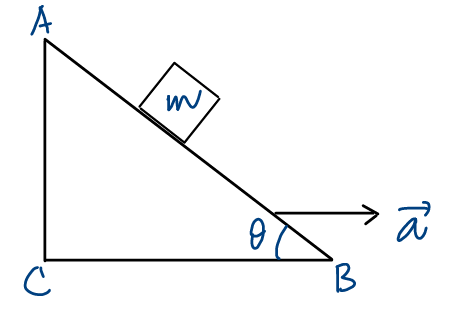# How To Solve NEET 2018 With A Smile – IV

### Let Us YOU Solve NEET 2018

We shall discuss some more Physics questions from NEET 2018. As usual, we shall help you to link them with the relevant theories. If required, you can study the theories from the video lectures referenced @ PhysicsAcademyOnline.com. Back into the question, you will solve it by your own effort. Lastly, you will confirm your answer from the video solution given in the blog.

Question 174:  A block of mass m is placed on a smooth inclined wedge ABC of inclination θ , as shown in the figure . The wedge is given an acceleration, a, towards the right. The relation between a and θ  for the block to remain stationary on the wedge is:(1) a = g /cosecθ           (2) a = g tanθ         (3) a = g cosθ        (4) a = g /sinθ

What is the effect of the accelerated motion of the wedge on the block? Subscribe to PhysicsAcademyOnline.com, and learn the basics from:  Chapter Name – Mechanics; Category –  Basic; Topic Name – Newton’s Laws of Motion; Video Name – Pseudo Force.

Show all the forces acting on the block in wedge-frame. What are their components, if any, along the incline AB? Brush up from the lecture: Chapter Name – Mechanics; Category –  Basic; Topic Name – Vectors; Video Name – Resolution of Vectors.

For what condition does the block remain stationary?

Question 175:  A student measured the diameter of a small steel ball using a screw gauge of least count 0.001 cm. The main scale reading is 5 mm, and zero of circular scale division coincides with 25 divisions above the reference level. If screw gauge has a zero error of -0.004 cm, the correct diameter of the ball is:

(1) 0.521 cm              (2) 0.529 cm                (3) 0.053 cm                (4) 0.525 cm

This question is related to measurement of physical quantities in the laboratory. A simple formula can do the trick as follows:

Actual length = main scale reading + least count x coinciding scale division – zero error

Pick your choice, and confirm from below.

Question 176:  The moment of the force, F = (4i +5j – 6k) N, applied at (2, 0, -3) m, about the point (2, -2, -2) m is given by:

(1) (- 8i – 4j – 7k) N-m      (2) (- 7i – 4j – 8k) N-m        (3) (- 7i – 8j – 4k) N-m       (4) (- 4ij – 8k) N-m

What is the formula for moment (or torque) of a force about an origin? Learn from this lecture: Chapter Name – Mechanics; Category –  Basic; Topic Name – Rotational Mechanics; Video Name – Torque of a Force Acting on a Particle.

What is the position vector, r, of the point of application of the force?  You might need this: Chapter Name – Mechanics; Category –  Basic; Topic Name – Vectors; Video Name –  Resolution of a position vector in space.

How do you calculate a vector product of two vectors? See similar here: Chapter Name – Mechanics; Category –  Basic; Topic Name – Vectors; Video Name –  Vector product of two vectors.

Question 180:  At what temperature will the rms speed of oxygen molecules become just sufficient for escaping the Earth’s atmosphere? Given the mass of oxygen molecule, m = 5.31 x 10-26 kg; Boltzmann’s constant, kB = 1.38 x 10-23 J/K.

(1) 2.51 x 104 K                  (2) 1.25 x 104 K                  (3) 5.02 x 104 K                   (4) 1.61 x 105 K

What is the formula for rms speed of the molecules of a gas? Follow the lecture:   Chapter Name – Heat and Thermodynamics; Category –  Basic; Topic Name – Kinetic Theory of Gases and Properties of Vapour; Video Name – Translational Kinetic Energy of a Gas.

What is the escape speed from Earth’s atmosphere? You are expected to remember its value. For detailed study, however, see: Chapter Name – Mechanics; Category –  Basic; Topic Name – Gravitation; Video Name – Escape Speed.

Use the condition given, and calculate the required temperature.